The Linux bash, or the command line, lets you perform both basic and complex arithmetic and boolean operations. The commands like expr, jot, bc and, factor etc, help you in finding optimal mathematical solutions to complex problems. In this article, we will describe these commands and present examples that will serve as a basis for you to move to more useful mathematical solutions.

We have run the commands and procedures mentioned in this article on a Ubuntu 18.04 LTS system.

We are using the Ubuntu command line, the Terminal, in order to perform all the mathematical operations. You can open the Terminal either through the system Dash or the Ctrl+Alt+T shortcut.

## The expr command

The expr or the expression command in Linux is the most commonly used command that is used to perform mathematical calculations. You can use this command to perform functions like addition, subtraction, multiplication, division, incrementing a value and, even comparing two values. In this section, we will describe the syntax for performing the above mentioned calculations and also present how you can use the expr command to perform productive maths in the Ubuntu command line.

You can add two or more numbers through the expr command as follows:

\$ expr number1 + number2

Example:

`\$ expr 100 + 10`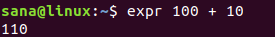### Subtraction

You can perform subtraction on two or more numbers through the expr command as follows:

\$ expr number1 – number2

Example:

`\$ expr 100 - 10`### Multiplication

You can perform multiplication of two or more numbers through the expr command as follows:

\$ expr number1 \* number2

Example:

`\$ expr 10 \* 10`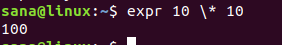Please note that following is the wrong syntax for number multiplication in the Linux command line as the command line uses simple asterisks as a reference to all files in the current directory.

\$ expr 10 * 10### Division

You can divide one number from the other through the expr command as follows:

\$ expr number1 / number2

Example:

`\$ expr 100 / 10`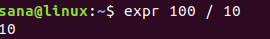### Increment a Variable

You can increment a variable in Linux by first defining it as follows:

`\$ count_variable=0`

And then, using the following expression, using the expr command, to perform the increment operation:

`\$ count_variable=`expr \$count_variable + 1``

Now when you echo the variable value through the following command, it will print the incremented value of the initially defined variable.

`\$ echo \$count_variable`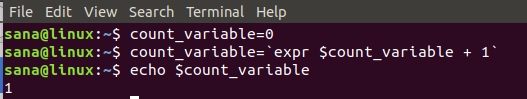### Comparison

Through the expr command, you can also check if a number is greater than another number or not.

\$ expr number1 \> number2

Example:

`\$ expr 20 \> 10`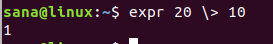The result value 1 indicates the first number is greater than the second one. Whereas, the result value 0 indicates the first number is not greater than the second one.

Example:

`\$ expr 10 \> 20`Through the expr command, you can also view if two number are equal or not by using the following syntax:

\$ expr number1 = number2

Examples:

`\$ expr 10 = 10`
`\$ expr 10 = 15`The result value 1 indicates that the first number is equal to the second one. Whereas, the result value 0 indicates the two numbers are not equal.

In the above examples, we can view from naked eyes that the two compared numbers are equal or not. So how is this operation useful?

Let us suppose that during a program, we want to know if the calculated average of a few numbers is equal to 10 or not. Here, we can use the best use of the expr command to compare the results of the “average” variable to 10 and perform further operations based on this result.

```\$ average=10
\$ expr \$average = 10```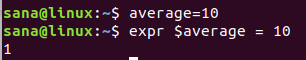### Making productive use of the expr command

Let us present an example where the expr command can be actually productive. In this example, we will use the expr command to calculate how many sweets a child gets when there are 10 children and 122 sweets. The program will also calculate the number of remaining sweets left after all children get an equal number of sweets.

```\$ children=10
\$ sweets=122
\$ PerChildShare=`expr \$sweets / \$children`
\$ RemainingSweets=`expr \$sweets - \$children \* \$PerChildShare`

echo \$PerChildShare
echo \$RemainingSweets```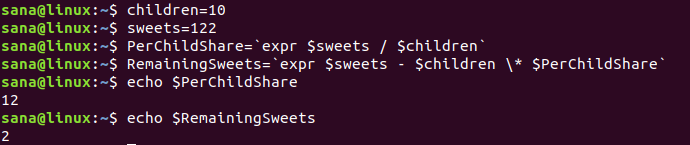The output perfectly displays that each child’s fair share of the take is 12, leaving 2 in the pot.

## The jot command

The Linux jot command helps you in creating a list of numbers based on the values that you provide as the starting number and the number of values you want to view after it. In this section, we will describe the syntax for performing calculations through jot and also present how you can use the jot command to perform productive maths in the Ubuntu command line.

You might first need to install Athena-jot in order to use the jot command on your Ubuntu.

Enter the following command as sudo as only an authorized person can add/remove and configure software on Ubuntu:

`\$ sudo apt install athena-jot`The system might prompt you with a Y/n option to confirm continuing with the installation. Please enter Y and then hit enter to continue after which the software will be installed on your system.

### Print x numbers after the number y

With the jot command, you can print x number of values after the number y. Use the following command to do so:

\$ jot x y

The following example will make the matters clear for you:

`\$ jot 5 1`Our example output displays a list of 5(x) values after the number 1(y).

### Reverse print x numbers after the number y till the number z

You can also use the jot command in order to reverse print x number of values after a number y, ending at the number z. Use the following syntax to do so:

\$ jot x y z

The following example will make the matters clear for you:

`\$ jot 9 10 2`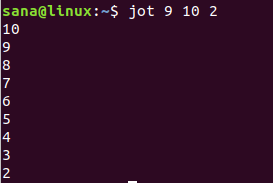Our example output reverse displays 9(x) values before the number 10(y), till the list reaches the number 2(z).

### Making productive use of the jot command

Lets us present an example where the jot command can be actually productive. In this example, we will use the jot command to print 15(value of x) days of January starting at day 1(value of y).

`\$ for i in `jot 15 1`; do echo January \$i; done`

Here is the output: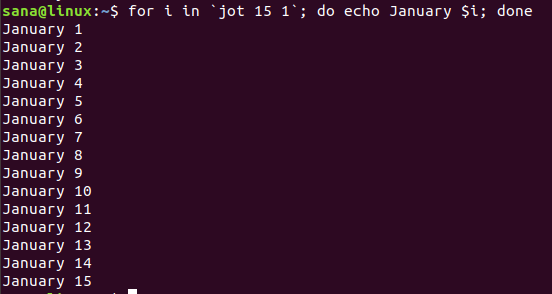We have used the for loop by using the output of jot command as the input value for the variable i.

## The factor command

As is clear by the name, the factor command in Linux is used to calculate the value of factors of a number we provide.

Syntax:

\$ factor number

Examples:In the above examples, you can see how the factor command prints multiple factors of a given number except 13, as the number 13 has no other factors than itself.

## The bc command

The bc command is very useful when performing complex operations in the bash. This command can also make comparisons, handle Booleans, and calculate square roots, sines, cosines, and tangents, etc. All you need to do is that you pipe the mathematical expression to the bc command as follows:

\$ echo “math_expression” | bc

The output then displays the result of the mathematical expression.

Example:

`\$ echo "10+10/2-(2*2)" | bc`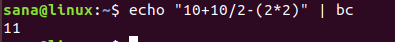### Calculating Pi

Since the bc command does not shy away from precision and the string you want to enter is pretty easy, you can use it to calculate the value of pi to your desired number of decimal points.

In the following example, we are printing the value of pi by specifying a scale value to round off decimal.

`\$ echo "scale=2; 4*a(1)" | bc -l`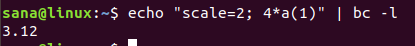Since the scale value is 2, the result of pi will be scaled to two decimal points.

`echo "scale=10; 4*a(1)" | bc -l`Since the scale value is 10, the result of pi will be scaled to ten decimal points.

## Performing Bash math

You can perform easy bash math by using a set of double parenthesis. You can perform both arithmetic and boolean operations in bash through this technique.

### Arithmetic

The following list of arithmetic operations can be performed in the Linux bash:

 +, – Addition, subtraction ++, — Increment, decrement *, / , % Multiplication, division, remainder ** Exponent value

Example 1:

```\$ ((x=10))
\$ (( e = e + 5 ))
\$ echo \$e```

Example 2:

```\$ ((x=10))
\$ ((x--))
\$ echo \$e```

Example 3:

```\$ ((x=10))
\$ ((x=x**2))
\$ echo \$e```

### Boolean and Logical

The bash math also allows you to use boolean and logical expressions within doubt parenthesis to perform mathematical calculations.

Example:

```\$ ((x=10)); ((y=5))

if (( x > y )); then echo \$x; fi```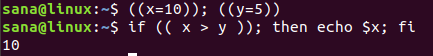This example compares two variables and outputs the value of x only if it is greater than y.

Through the command syntax and examples presented in this article, you are now capable of performing basic math operations through Linux bash. This will serve as a basis for you to perform more complex operations such as using bash scripts to write programs. Moreover, you can also perform interactive maths where you can input values from the user, process the input and then output results based on those values.

How to do Basic Math in Linux Command Line#### Karim Buzdar

About the Author: Karim Buzdar holds a degree in telecommunication engineering and holds several sysadmin certifications. As an IT engineer and technical author, he writes for various web sites. You can reach Karim on LinkedIn